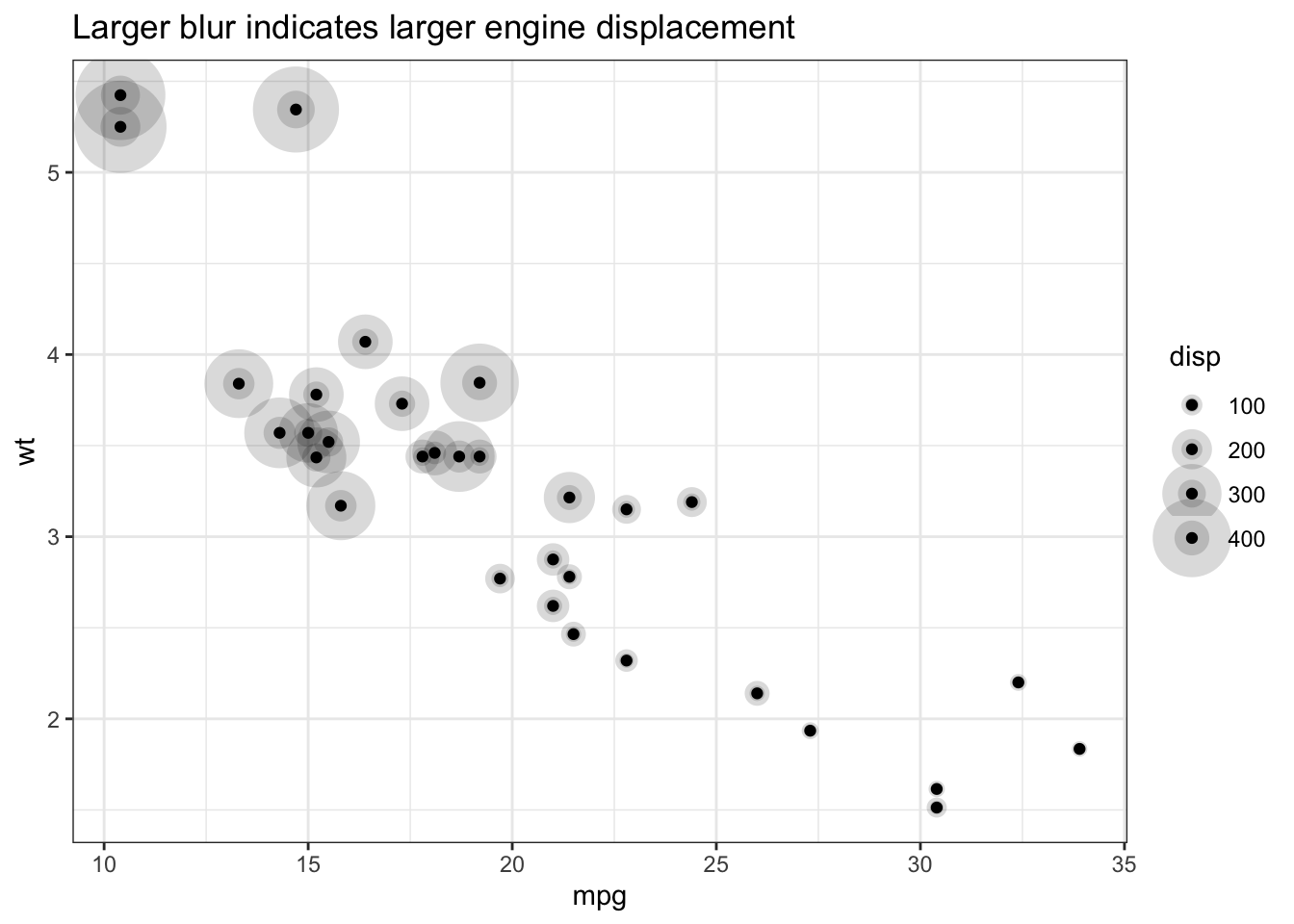# ggblur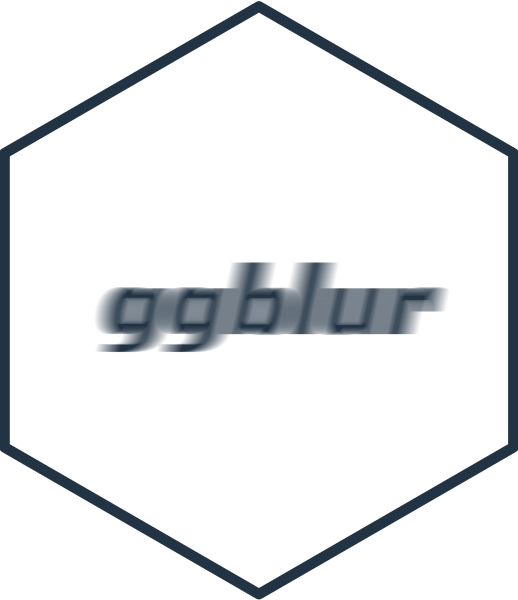`ggblur` provides `geom_point_blur()` for use in `ggplot` - this geom allows you to control the blurriness of the drawn points, but is otherwise identical to the standard `geom_point()`.

#### What’s in the box?

• `geom_point_blur()`
• same as `geom_point()` but now accepts `blur_size` as an aesthetic
• also allows for control of the smoothness of the blur (`blur_steps`) and the maximum opacity (`blur_alpha`)
• `scale_blur_size_continuous()`, `scale_blur_size_discrete()` and `scale_blur_size_manual()` for controlling `blur_size` when used as a mapped aesthetic/

#### Similar packages

• ggecho is an earlier experiment of mine from 2019 where I echo components using a custom stat `stat_echo`. This solution works across multiple geoms, but didn’t allow for adjusting the echo for individual points.
• ggfx adds blur at the layer level i.e.  no individual control over the amount of blur for each point.

#### How it works

Blur is simulated by keeping the original point, and drawing a sequence of larger, faded points behind it (illustrated below).

The number of points rendered is controlled by `blur_steps` and the distance to which the blur extends beyond the edge of the original point is controlled by `blur_size`.

Note: Paul Murrell has some experiments to add radial gradients to grid graphics (see his github) and if this becomes part of grid it may be a better method of simulating blur.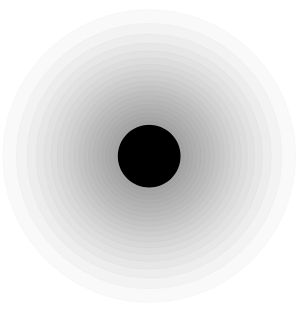## Installation

You can install the development version from GitHub with:

``````# install.packages("devtools")
devtools::install_github("coolbutuseless/ggblur")``````

## Example 1 - constant `blur_size`

When not used as a mapped aesthetic within `aes()`, the same `blur_size` is applied to every point.

``````library(ggplot2)
library(ggblur)

ggplot(mtcars) +
geom_point_blur(aes(mpg, wt), blur_size = 10) +
theme_bw() +
labs(title = "Same blur for each point")``````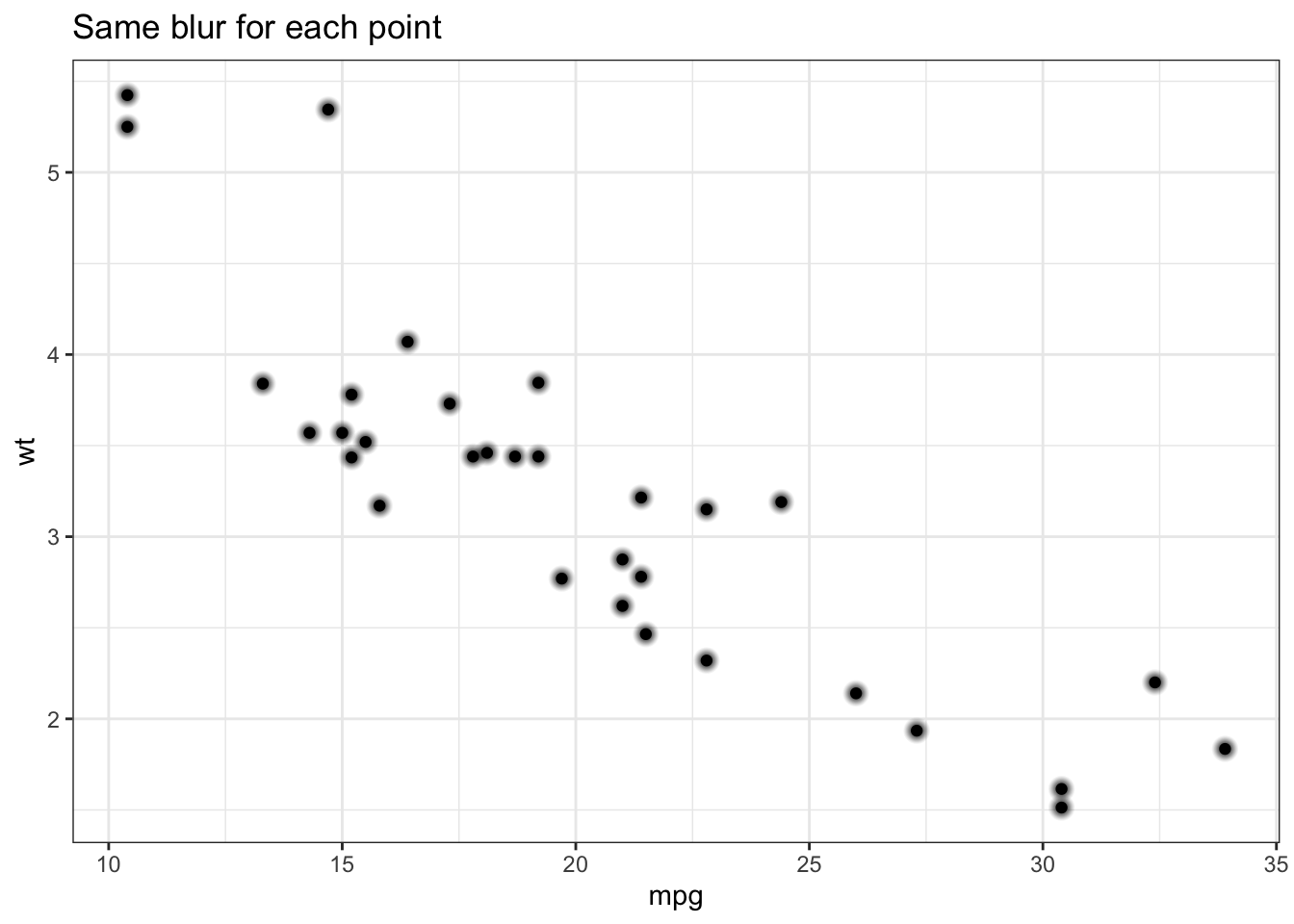## Example 2 - mapping `blur_size` as an aesthetic

When used as a mapped aesthetic within `aes()`, the `blur_size` is calculated individually for each point.

``````library(ggplot2)
library(ggblur)

ggplot(mtcars) +
geom_point_blur(aes(mpg, wt, blur_size = disp)) +
theme_bw() +
labs(title = "Larger blur indicates larger engine displacement")``````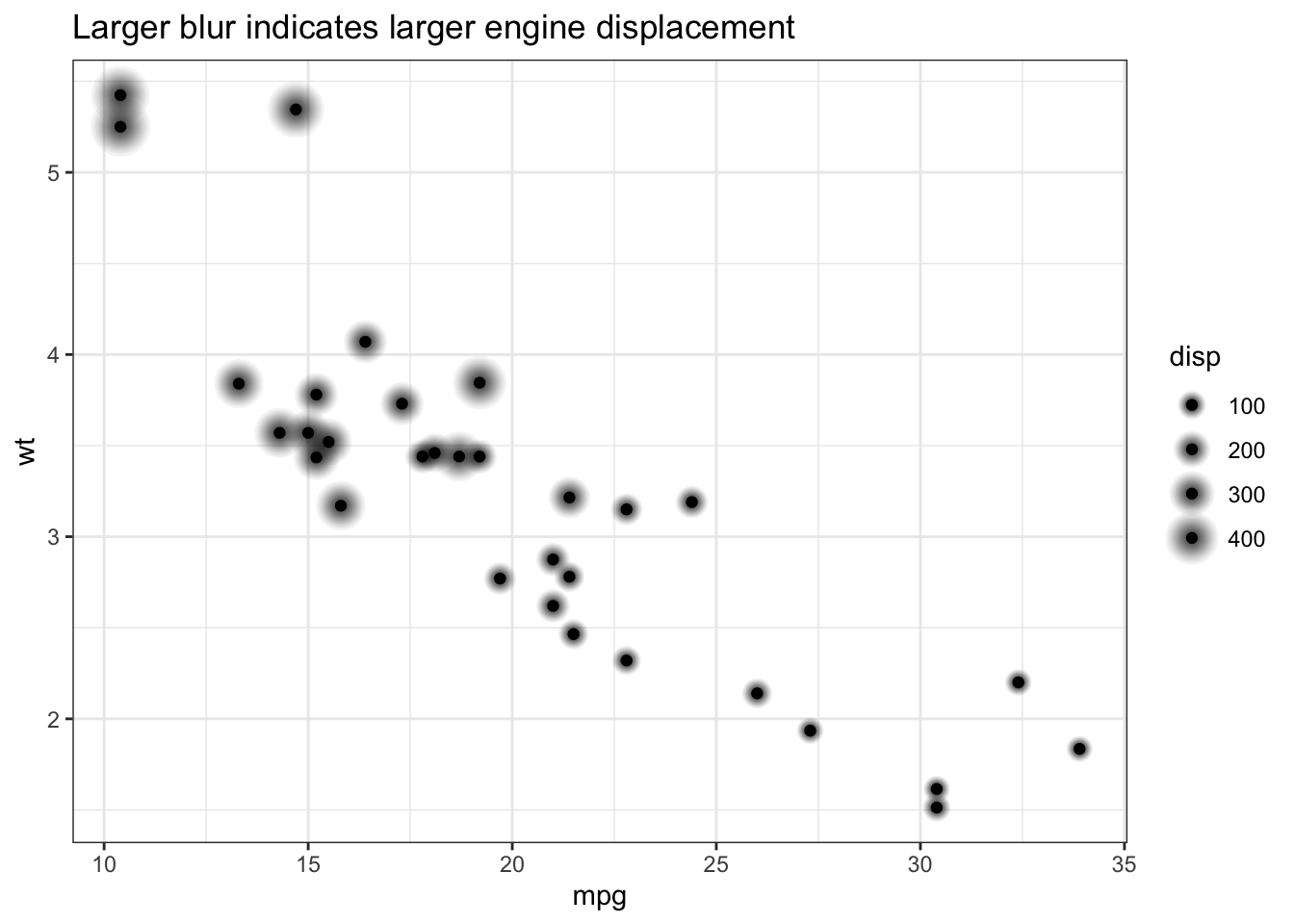## Example 3 - control over blur parameters

`blur_alpha`, `blur_steps` and `scale_blue_size_continuous/discrete/manual()` can be used to further customise the appearance.

``````ggplot(mtcars) +
geom_point_blur(aes(mpg, wt, blur_size = disp), blur_alpha = 0.3, blur_steps = 2) +
scale_blur_size_continuous(range = c(1, 15)) +
theme_bw() +
labs(title = "Larger blur indicates larger engine displacement")``````